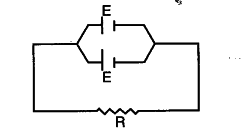# Two identical cells, each of emf E, having negligible internal resistance, are connected in parallel with each other across an external resistance R. What is the current through this resistance?

As the internal resistance is negligible, so the total resistance = R.
Potential difference across the Resistance = E.So, current through the resistance = I=E/R